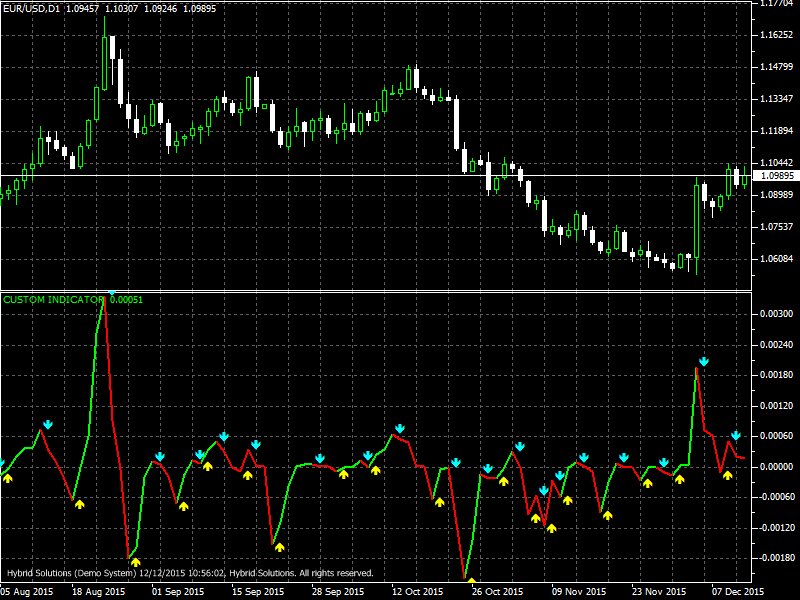# VQ

Free

In stock

VQ (Volatility Quality) is a VertexFX client side indicator. Volatility Quality is an indicator used for entering the market in the direction of the current trend. The indicator consists of trend line and generates signals to buy and sell in the form of arrows of yellow and blue.

Signals to buy are displayed as yellow arrows and blue arrows for sell signals. In an uptrend market the line of the indicator is displayed in green, and in the downtrend the line is desplayed in red. To calculate the values of the indicator, we use Moving Averages (MA) values separately for the prices of Open, High, Low and Close. The basic formula for calculating the indicator:

SKU: 888 Categories: ,VQ (Volatility Quality) is a VertexFX client side indicator. Volatility Quality is an indicator used for entering the market in the direction of the current trend. The indicator consists of trend line and generates signals to buy and sell in the form of arrows of yellow and blue.

Signals to buy are displayed as yellow arrows and blue arrows for sell signals. In an uptrend market the line of the indicator is displayed in green, and in the downtrend the line is desplayed in red. To calculate the values of the indicator, we use Moving Averages (MA) values separately for the prices of  Open, High, Low and Close. The basic formula for calculating the indicator:
TR = Abs(((MA_Close(i)-MA_Close(i-1))/Max(MA_High(i)-MA_Low(i),Max(MA_High(i)-MC1(i),MC1(i)-MA_Low(i)))+(MA_Close(i)-MA_Open(i))/(MA_High(i)-MA_Low(i)))*0.5)*((MA_Close(i)-MA_Close(i-1)+(MA_Close(i)-MA_Open(i)))*0.5) MA-moving Average

Volatility quality indicator is advised to be used with additional filter indicators, for best performance of the indicator. The Volatility Quality indicator is most suitable for major pairs, on H1 and H4 time frames.

The input parameters of the indicator:
-Length (default = 5) – affects the frequency and accuracy of the signals;
-Method (default = 3) – a method of smoothing
-Smoothing (default = 1) – smoothing degree
-Filter (default = 5) – filtration, the higher the value, the lower the signal and they are more precise on trends
-Count Bars (default = 400) – number of bars in history, which is displayed